# Program for all operations on circular linked list in C

• Difficulty Level : Easy
• Last Updated : 18 Aug, 2021

In a Circular linked list, every element has a link to its next element in the sequence and the last element has a link to the first element. A circular linked list is similar to the singly linked list except that the last node points to the first node. Below is the image to illustrate the same: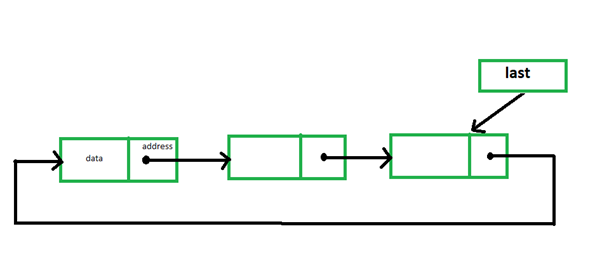Some common operations of a circular linked list are implemented below:

Insertion at the beginning: Inserting a new node as the first node. The next pointer of last will point to this node and this new node will point to the previous first node.

## C

 `// C program for the above operation` `#include ` `#include `   `// Structure of a linked list node` `struct` `node {` `    ``int` `info;` `    ``struct` `node* next;` `};`   `// Pointer to last node in the list` `struct` `node* last = NULL;`   `// Function to insert a node in the` `// starting of the list` `void` `insertAtFront()` `{` `    ``// Stores the number to be inserted` `    ``int` `data;`   `    ``// Initialize a new node` `    ``struct` `node* temp;` `    ``temp = (``struct` `node*)``malloc``(``sizeof``(``struct` `node));`   `    ``// Input data` `    ``printf``(``"\nEnter data to be "` `           ``"inserted: \n"``);` `    ``scanf``(``"%d"``, &data);`   `    ``// If the new node is the only` `    ``// node in the list` `    ``if` `(last == NULL) {` `        ``temp->info = data;` `        ``temp->next = temp;` `        ``last = temp;` `    ``}`   `    ``// Else last node contains the` `    ``// reference of the new node and` `    ``// new node contains the reference` `    ``// of the previous first node` `    ``else` `{` `        ``temp->info = data;` `        ``temp->next = last->next;`   `        ``// last node now has reference` `        ``// of the new node temp` `        ``last->next = temp;` `    ``}` `}`   `// Function to print the list` `void` `viewList()` `{` `    ``// If list is empty` `    ``if` `(last == NULL)` `        ``printf``(``"\nList is empty\n"``);`   `    ``// Else print the list` `    ``else` `{` `        ``struct` `node* temp;` `        ``temp = last->next;`   `        ``// While first node is not` `        ``// reached again, print,` `        ``// since the list is circular` `        ``do` `{` `            ``printf``(``"\nData = %d"``, temp->info);` `            ``temp = temp->next;` `        ``} ``while` `(temp != last->next);` `    ``}` `}`   `// Driver Code` `int` `main()` `{` `    ``// Function Call` `    ``insertAtFront();` `    ``insertAtFront();` `    ``insertAtFront();`   `    ``// Print list` `    ``viewList();`   `    ``return` `0;` `}`

Output: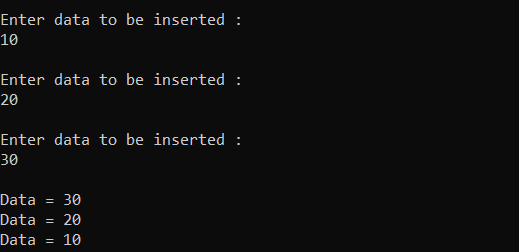Insertion at the end: Inserting a new node as the last node. The next pointer of last will point to this node and this new node will point to the first node.

## C

 `// C program for the above operation` `#include ` `#include `   `// Structure of a linked list node` `struct` `node {` `    ``int` `info;` `    ``struct` `node* next;` `};`   `// Pointer to last node in the list` `struct` `node* last = NULL;`   `// Function to add a new node at the` `// end of the list` `void` `addatlast()` `{` `    ``// Stores number to be inserted` `    ``int` `data;`   `    ``// Initialize a new node` `    ``struct` `node* temp;` `    ``temp = (``struct` `node*)``malloc``(``sizeof``(``struct` `node));`   `    ``// Input data` `    ``printf``(``"\nEnter data to be "` `           ``"inserted : \n"``);` `    ``scanf``(``"%d"``, &data);`   `    ``// If the new node is the` `    ``// only node in the list` `    ``if` `(last == NULL) {` `        ``temp->info = data;` `        ``temp->next = temp;` `        ``last = temp;` `    ``}`   `    ``// Else the new node will be the` `    ``// last node and will contain` `    ``// the reference of head node` `    ``else` `{` `        ``temp->info = data;` `        ``temp->next = last->next;` `        ``last->next = temp;` `        ``last = temp;` `    ``}` `}`   `// Function to print the list` `void` `viewList()` `{` `    ``// If list is empty` `    ``if` `(last == NULL)` `        ``printf``(``"\nList is empty\n"``);`   `    ``// Else print the list` `    ``else` `{` `        ``struct` `node* temp;` `        ``temp = last->next;` `        ``do` `{` `            ``printf``(``"\nData = %d"``,` `                   ``temp->info);` `            ``temp = temp->next;` `        ``} ``while` `(temp != last->next);` `    ``}` `}`   `// Driver Code` `int` `main()` `{` `    ``// Function Call` `    ``addatlast();` `    ``addatlast();` `    ``addatlast();`   `    ``// Print list` `    ``viewList();`   `    ``return` `0;` `}`

Output: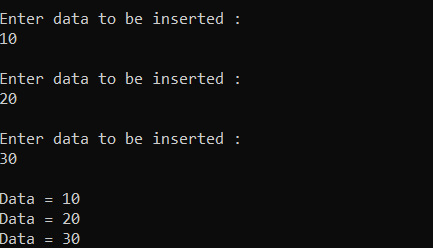Insertion after a specific element: Below is the program to insert a node after a specified node in the linked list.

## C

 `// C program for the above operation` `#include ` `#include `   `// Structure of a linked list node` `struct` `node {` `    ``int` `info;` `    ``struct` `node* next;` `};`   `// Pointer to last node in list` `struct` `node* last = NULL;`   `// Function to add a new node` `// at the end of the list` `void` `addatlast()` `{` `    ``// Stores number to be inserted` `    ``int` `data;`   `    ``// Initialize a new node` `    ``struct` `node* temp;` `    ``temp = (``struct` `node*)``malloc``(``sizeof``(``struct` `node));`   `    ``// Input data` `    ``printf``(``"\nEnter data to be inserted : \n"``);` `    ``scanf``(``"%d"``, &data);`   `    ``// If the new node is the` `    ``// only node in the list` `    ``if` `(last == NULL) {` `        ``temp->info = data;` `        ``temp->next = temp;` `        ``last = temp;` `    ``}`   `    ``// Else the new node will be the` `    ``// last node and will contain` `    ``// the reference of head node` `    ``else` `{` `        ``temp->info = data;` `        ``temp->next = last->next;` `        ``last->next = temp;` `        ``last = temp;` `    ``}` `}`   `// Function to insert after any` `// specified element` `void` `insertafter()` `{` `    ``// Stores data and element after` `    ``// which new node is to be inserted` `    ``int` `data, value;`   `    ``// Initialize a new node` `    ``struct` `node *temp, *n;`   `    ``// Input data` `    ``printf``(``"\nEnter number after which"` `           ``" you want to enter number: \n"``);` `    ``scanf``(``"%d"``, &value);` `    ``temp = last->next;`   `    ``do` `{`   `        ``// Element after which node is` `        ``// to be inserted is found` `        ``if` `(temp->info == value) {` `            ``n = (``struct` `node*)``malloc``(``sizeof``(``struct` `node));`   `            ``// Input Data` `            ``printf``(``"\nEnter data to be"` `                   ``" inserted : \n"``);` `            ``scanf``(``"%d"``, &data);` `            ``n->info = data;` `            ``n->next = temp->next;` `            ``temp->next = n;`   `            ``// If temp is the last node` `            ``// so now n will become the` `            ``// last node` `            ``if` `(temp == last)` `                ``last = n;` `            ``break``;` `        ``}` `        ``else` `            ``temp = temp->next;` `    ``} ``while` `(temp != last->next);` `}`   `// Function to print the list` `void` `viewList()` `{` `    ``// If list is empty` `    ``if` `(last == NULL)` `        ``printf``(``"\nList is empty\n"``);`   `    ``// Else print the list` `    ``else` `{` `        ``struct` `node* temp;` `        ``temp = last->next;` `        ``do` `{` `            ``printf``(``"\nData = %d"``,` `                   ``temp->info);` `            ``temp = temp->next;` `        ``} ``while` `(temp != last->next);` `    ``}` `}`   `// Driver Code` `int` `main()` `{` `    ``// Initialize the list` `    ``addatlast();` `    ``addatlast();` `    ``addatlast();`   `    ``// Function Call` `    ``insertafter();`   `    ``// Print list` `    ``viewList();`   `    ``return` `0;` `}`

Output: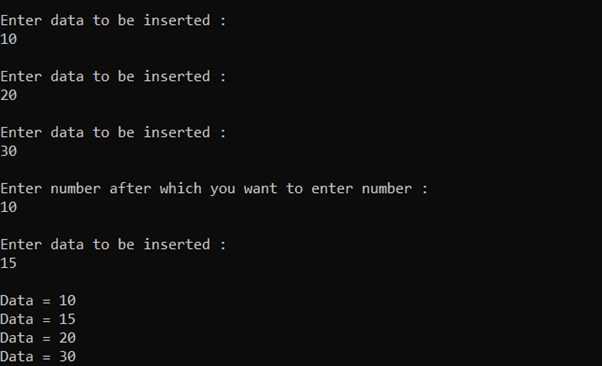Delete first element: Deleting the first node of the linked list. For this, the next pointer of last will point to the second node of the linked list.  Below is the program for the same:

## C

 `// C program for the above operation` `#include ` `#include `   `// Structure of a linked list node` `struct` `node {` `    ``int` `info;` `    ``struct` `node* next;` `};`   `// Pointer to last node in list` `struct` `node* last = NULL;`   `// Function to add a new node` `// at the end of the list` `void` `addatlast()` `{` `    ``// Stores number to be inserted` `    ``int` `data;`   `    ``// Initialize a new node` `    ``struct` `node* temp;` `    ``temp = (``struct` `node*)``malloc``(``sizeof``(``struct` `node));`   `    ``// Input data` `    ``printf``(``"\nEnter data to be"` `           ``" inserted: \n"``);` `    ``scanf``(``"%d"``, &data);`   `    ``// If the new node is the only` `    ``// node in the list` `    ``if` `(last == NULL) {` `        ``temp->info = data;` `        ``temp->next = temp;` `        ``last = temp;` `    ``}`   `    ``// Else the new node will be the` `    ``// last node and will contain` `    ``// the reference of head node` `    ``else` `{` `        ``temp->info = data;` `        ``temp->next = last->next;` `        ``last->next = temp;` `        ``last = temp;` `    ``}` `}`   `// Function to delete the first` `// element of the list` `void` `deletefirst()` `{` `    ``struct` `node* temp;`   `    ``// If list is empty` `    ``if` `(last == NULL)` `        ``printf``(``"\nList is empty.\n"``);`   `    ``// Else last node now contains` `    ``// reference of the second node` `    ``// in the list because the` `    ``// list is circular` `    ``else` `{` `        ``temp = last->next;` `        ``last->next = temp->next;` `        ``free``(temp);` `    ``}` `}`   `// Function to print the list` `void` `viewList()` `{` `    ``// If list is empty` `    ``if` `(last == NULL)` `        ``printf``(``"\nList is empty\n"``);`   `    ``// Else print the list` `    ``else` `{` `        ``struct` `node* temp;` `        ``temp = last->next;` `        ``do` `{` `            ``printf``(``"\nData = %d"``,` `                   ``temp->info);` `            ``temp = temp->next;` `        ``} ``while` `(temp != last->next);` `    ``}` `}`   `// Driver Code` `int` `main()` `{` `    ``// Initialize the list` `    ``addatlast();` `    ``addatlast();` `    ``addatlast();`   `    ``// Function Call` `    ``deletefirst();`   `    ``// Print list` `    ``viewList();`   `    ``return` `0;` `}`

Output: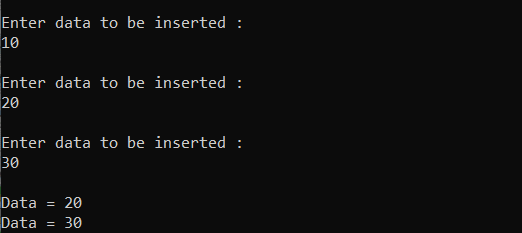Delete the last element: Deleting the last node of the linked list. For this, the second last node will point to the first node of the list. Below is the program for the same:

## C

 `// C program for the above operation` `#include ` `#include `   `// Structure of a linked list node` `struct` `node {` `    ``int` `info;` `    ``struct` `node* next;` `};`   `// Pointer to last node in list` `struct` `node* last = NULL;`   `// Function to add a new node` `// at the end of the list` `void` `addatlast()` `{` `    ``// Stores number to be inserted` `    ``int` `data;`   `    ``// Initialize a new node` `    ``struct` `node* temp;` `    ``temp = (``struct` `node*)``malloc``(``sizeof``(``struct` `node));`   `    ``// Input data` `    ``printf``(``"\nEnter data to be inserted : \n"``);` `    ``scanf``(``"%d"``, &data);`   `    ``// If the new node is the only` `    ``// node in the list` `    ``if` `(last == NULL) {` `        ``temp->info = data;` `        ``temp->next = temp;` `        ``last = temp;` `    ``}`   `    ``// Else the new node will be` `    ``// last node and will contain` `    ``// the reference of head node` `    ``else` `{` `        ``temp->info = data;` `        ``temp->next = last->next;` `        ``last->next = temp;` `        ``last = temp;` `    ``}` `}`   `// Function to delete the last node` `// in the list` `void` `deletelast()` `{` `    ``struct` `node* temp;`   `    ``// If list is empty` `    ``if` `(last == NULL)` `        ``printf``(``"\nList is empty.\n"``);`   `    ``temp = last->next;`   `    ``// Traverse the list till` `    ``// the second last node` `    ``while` `(temp->next != last)` `        ``temp = temp->next;`   `    ``// Second last node now contains` `    ``// the reference of the first` `    ``// node in the list` `    ``temp->next = last->next;` `    ``last = temp;` `}`   `// Function to print the list` `void` `viewList()` `{` `    ``// If list is empty` `    ``if` `(last == NULL)` `        ``printf``(``"\nList is empty\n"``);`   `    ``// Else print the list` `    ``else` `{` `        ``struct` `node* temp;` `        ``temp = last->next;` `        ``do` `{` `            ``printf``(``"\nData = %d"``,` `                   ``temp->info);` `            ``temp = temp->next;` `        ``} ``while` `(temp != last->next);` `    ``}` `}`   `// Driver Code` `int` `main()` `{` `    ``// Initialize the list` `    ``addatlast();` `    ``addatlast();` `    ``addatlast();`   `    ``// Function Call` `    ``deletelast();`   `    ``// Print the list` `    ``viewList();`   `    ``return` `0;` `}`

Output: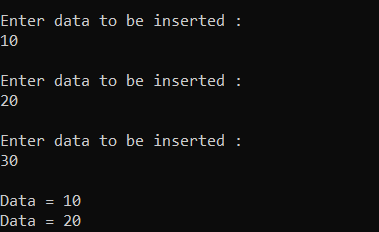Delete at a given position: Delete an element from a specified position in the linked list. Below is the program for the same:

## C

 `// C program for the above operation` `#include ` `#include `   `// Structure of a linked list node` `struct` `node {` `    ``int` `info;` `    ``struct` `node* next;` `};`   `// Pointer to last node in list` `struct` `node* last = NULL;`   `// Function to add a new node` `// at the end of the list` `void` `addatlast()` `{` `    ``// Stores number to be inserted` `    ``int` `data;`   `    ``// Initialize a new node` `    ``struct` `node* temp;` `    ``temp = (``struct` `node*)``malloc``(``sizeof``(``struct` `node));`   `    ``// Input data` `    ``printf``(``"\nEnter data to be inserted : \n"``);` `    ``scanf``(``"%d"``, &data);`   `    ``// If the new node is the` `    ``// only node in the list` `    ``if` `(last == NULL) {` `        ``temp->info = data;` `        ``temp->next = temp;` `        ``last = temp;` `    ``}`   `    ``// Else the new node will be` `    ``// last node and will contain` `    ``// the reference of head node` `    ``else` `{` `        ``temp->info = data;` `        ``temp->next = last->next;` `        ``last->next = temp;` `        ``last = temp;` `    ``}` `}`   `// Function to delete an element` `// at a specified index in the list` `void` `deleteAtIndex()` `{` `    ``// Stores the index at which` `    ``// the element is to be deleted` `    ``int` `pos, i = 1;` `    ``struct` `node *temp, *position;` `    ``temp = last->next;`   `    ``// If list is empty` `    ``if` `(last == NULL)` `        ``printf``(``"\nList is empty.\n"``);`   `    ``// Else` `    ``else` `{`   `        ``// Input Data` `        ``printf``(``"\nEnter index : "``);` `        ``scanf``(``"%d"``, &pos);`   `        ``// Traverse till the node to` `        ``// be deleted is reached` `        ``while` `(i <= pos - 1) {` `            ``temp = temp->next;` `            ``i++;` `        ``}`   `        ``// After the loop ends, temp` `        ``// points at a node just before` `        ``// the node to be deleted`   `        ``// Reassigning links` `        ``position = temp->next;` `        ``temp->next = position->next;`   `        ``free``(position);` `    ``}` `}`   `// Function to print the list` `void` `viewList()` `{` `    ``// If list is empty` `    ``if` `(last == NULL)` `        ``printf``(``"\nList is empty\n"``);`   `    ``// Else print the list` `    ``else` `{` `        ``struct` `node* temp;` `        ``temp = last->next;` `        ``do` `{` `            ``printf``(``"\nData = %d"``, temp->info);` `            ``temp = temp->next;` `        ``} ``while` `(temp != last->next);` `    ``}` `}`   `// Driver Code` `int` `main()` `{` `    ``// Initialize the list` `    ``addatlast();` `    ``addatlast();` `    ``addatlast();`   `    ``// Function Call` `    ``deleteAtIndex();`   `    ``// Print the list` `    ``viewList();`   `    ``return` `0;` `}`

Output: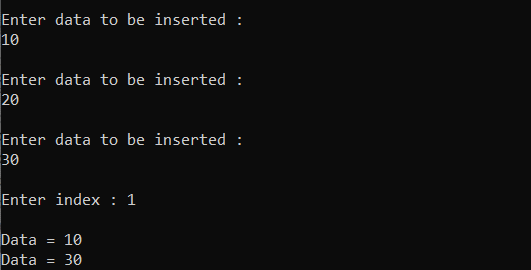My Personal Notes arrow_drop_up
Recommended Articles
Page :